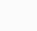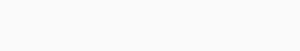# WMI Preliminary Round 2021 [Grade 6A]

World Mathematics Invitational (WMI) is the first international competition founded by Taiwan. It gathers institutes and organizations worldwide that make efforts in promoting and popularizing mathematics. Through interacting with other math-loving students that represent their countries, students can expand their worldview, experience different cultures, and thus their horizon as well as their future will be broaden. (sc : http://www.wminv.org/)

Berikut ini soal dan solusi WMI grade 6A tahun 2021

1. If $$\frac{3}{4}𝑥 =\frac{7}{12}$$, find $$𝑥$$

(A) $$\frac{7}{9}$$
(B) $$\frac{9}{7}$$
(C) $$\frac{7}{8}$$
(D) $$\frac{3}{7}$$

$$\frac{3}{4}𝑥 =\frac{7}{12}$$
$$⇒ 𝑥 =\frac{7}{12}×\frac{4}{3}=\frac{7}{9}$$

2. Compute $$(6\frac{6}{7}− 3\frac{9}{13}) ÷ 2\frac{7}{13}$$

(A) $$1\frac{19}{91}$$
(B) $$1\frac{17}{77}$$
(C) $$1\frac{17}{91}$$
(D) $$1\frac{19}{77}$$

$$(6\frac{6}{7}− 3\frac{9}{13}) ÷ 2\frac{7}{13}$$
$$= (6 − 3 +\frac{6}{7}−\frac{9}{13}) ÷\frac{33}{13}$$
$$= (3 +\frac{78 − 63}{91}) ×\frac{13}{33}$$
$$= (3 +\frac{15}{91}) ×\frac{13}{33}$$
$$= (\frac{288}{91}) ×\frac{13}{33}$$
$$=\frac{96}{77}=1\frac{19}{77}$$

3. Suppose the 6-digit number 21578◻ is divisible by 12, what is the number in the square?

(A) 8
(B) 6
(C) 4
(D) 2

Syarat bilangan habis dibagi 12 adalah habis dibagi 4 dan 3.

• Syarat habis dibagi 4 adalah 2 angka terakhir habis dibagi 4, dua angka terakhir dari 21578◻ adalah 8◻, nilai ◻ yang memenuhi adalah 4 atau 8.
• Syarat habis dibagi 3 adalah jumlah angkanya habis dibagi 3
2 + 1 + 5 + 7 + 8 +◻= 23 +◻
Bilangan kelipatan 3 terdekat dari 23 adalah 24, 27, atau 30, nilai ◻ yang memenuhi adalah 1, 4 atau 7.

4. Divide the product of 36158 and 273 by 7, what is the remainder?

(A) 0
(B) 1
(C) 2
(D) 3

$$36158 × 273\; 𝑚𝑜𝑑\; 7 ≡ 3 × 0\; 𝑚𝑜𝑑\; 7 ≡ 0$$

atau karena 273 habis dibagi 7 maka hasil kali kedua bilangan juga habis dibagi 7. Jadi sisa hasil kali kedua bilangan jika dibagi 7 adalah 0

5. Two cars A and B drive on the same road. If their time ratio is 3：4, what is their speed ratio?

(A) 3 : 4
(B) 4 : 3
(C) 3 : 7
(D) 7 : 3

Kecepatan berbanding terbalik dengan waktu, Kecepatan semakin besar waktunya semakin singkat demikian sebaliknya. Jadi jika perbandingan waktu mobil A dan B pas
sampai di tujuan adalah 3 : 4, maka perbandingan kecepatan mobil A dan B adalah kebalikannya yaitu 4 : 3.

6. In the class, the number of girls is half the number of boys. If the average weight for boys is 35kg, the average weight for girls is 32kg, find the average weight for the class in kg.
(A) 32.5
(B) 33
(C) 33.5
(D) 34

Misalkan banyak anak perempuan adalah $$G$$ dan banyak anak laki-laki adalah $$B$$. Diketahui
$$𝐺 =\frac{1}{2}𝐵 ⇒ 𝐵 = 2𝐺$$. Berat rata-rata anak laki-laki adalah $$35$$ kg, berat rata-rata anak perempuan adalah $$32$$ kg. Rata-rata kelas adalah

$$\frac{35𝐵 + 32𝐺}{𝐵 + 𝐺}=\frac{35(2𝐺) + 32𝐺}{2𝐺 + 𝐺}=\frac{70𝐺 + 32𝐺}{3𝐺}=\frac{102𝐺}{3𝐺}= 34$$

Jadi rata-rata kelas adalah $$34$$ kg

7. Make the handspin. What is the probability that it stops at an even number?(A) $$\frac{1}{2}$$
(B) $$\frac{1}{3}$$
(C) $$\frac{1}{4}$$
(D) $$\frac{2}{3}$$

8) Given that $$a, b, c,$$ and d aren’t $$0$$. Set $$P＝a÷b×c÷d$$, which expression below equals $$P$$?

(A) $$a×b÷d÷c$$
(B) $$a×d÷c÷b$$
(C) $$a÷(b÷c)÷d$$
(D) $$a÷b÷c÷d$$

9.  [A] represents the number of natural number A’s factors. For example, 4 has three factors 1, 2, and 4, so it can be written as ＝3. Find ÷.

(A) 1
(B) 2
(C) 3
(D) 4

10. $$\frac{1}{5}$$ of number $$A$$ equals $$\frac{1}{4}$$ of number $$B$$. $$25\%$$ of number $$A$$ equals $$20\%$$ of number $$C$$. Compare the three numbers $$A, B,$$ and $$C$$. Which option below is correct?

(A) A＞B＞C
(B) C＞B＞A
(C) A＞C＞B
(D) C＞A＞B

11. Use 3 sticks to form a triangle. Place the sticks as below. How many sticks are needed to form 100 triangles?(A) 3×100
(B) 3×50＋50＋1
(C) 2×100＋1
(D) 3×100－100

Satu segitiga $$⇒ 3$$ stik
Dua segitiga $$⇒ 5$$ stik
Tiga segitiga $$⇒ 7$$ stik

$$n$$ segitiga $$⇒ 2𝑛 + 1$$ stik
Jadi 100 segitiga membutuhkan $$2(100) + 1 = 201$$ stik

12. There are three different squares whose side lengths are $$a, b,$$ and $$c$$, respectively. If $$a, b$$, and $$c$$ are integers, and the total area of the three squares is $$202$$, find $$𝑎𝑏𝑐$$.

(A) 48
(B) 84
(C) 96
(D) 120

$$𝑎^2 + 𝑏^2 + 𝑐^2 = 202$$

Bilangan kuadrat kurang dari $$200$$ yaitu $$\{1, 4, 9, 16, 25, 36, 49, 64, 81, 100, 121, 144, 169, 196\}$$
Dengan melakukan observasi nilai diperoleh

$$9 + 49 + 144 = 202$$
$$32 + 72 + 122 = 202$$

Nilai $$𝑎, 𝑏$$ dan $$c$$ yang memenuhi adalah $$3, 7$$ dan $$12$$. Jadi nilai $$𝑎𝑏𝑐$$ adalah $$3(7)(12)=252$$

13. Use a 32 long iron wire to make a rectangle. If its length and width are primes, how many different values does its area have?

(A) 6
(B) 4
(C) 3
(D) 2

14) Given a 3-digit number. Divide it by 3, and the remainder is 1. Divide it by 4, and the remainder is 1. Find the largest possible value of this number.

(A) 998
(B) 997
(C) 996
(D) 995

Misalkan bilangan $$3$$ angka tersebut adalah $$𝑁$$ Karena $$N$$ dibagi $$3$$ atau $$4$$ bersisa $$1$$ maka nilai $$N$$ jika dibagi $$12$$ bersisa $$1$$ atau dapat ditulis

$$𝑁 = 12𝑘 + 1$$

Karena yang dicari adalah bilangan $$3$$ digit terbesar maka cari nilai k sehingga jika dikali dengan $$12$$ mendekati $$1000$$, nilai $$k$$ yang memenuhi adalah $$83$$. Jadi

$$𝑁 = 12𝑘 + 1 = 12(83) + 1 = 996 + 1 = 997$$

15. Use four identical rectangles and a small square (as shown on the right) to form a large square whose area is 49 m². If the area of the small square is 4 m², find the length of the shorter side of the rectangle in m.(A) 2
(B) 2.3
(C) 2.4
(D) 2.5Diperoleh persamaan

$$𝑝 + 𝑙 = 7$$
$$𝑝 − 𝑙 = 2$$

Kurangkan kedua persamaan, diperoleh

$$2𝑙 = 5 ⇒ 𝑙 =\frac{5}{2}= 2,5$$

Jadi panjang sisi terpendeknya adalah $$2,5$$